Courses

# GATE Past Year Questions: Kinematics of Mechanisms Notes | EduRev

## Mechanical Engineering : GATE Past Year Questions: Kinematics of Mechanisms Notes | EduRev

The document GATE Past Year Questions: Kinematics of Mechanisms Notes | EduRev is a part of the Mechanical Engineering Course Theory of Machines (TOM).
All you need of Mechanical Engineering at this link: Mechanical Engineering

Try yourself:For the planar mechanism shown in figure select the most appropriate choice for the motion of link 2 when link 4 is moved upwards.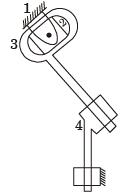Try yourself:For the audio Cassette mechanism shown in figure given below where is the instantaneous centre of rotation (P) of the two spools?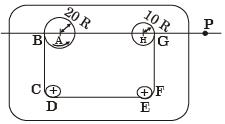Try yourself:The number of inversions for a slider crank mechanism is



Try yourself:Match the items in columns I and II.
Column-I
P. Higher kinematic pair
Q. Lower kinematic pair
R. Quick return mechanism
Column-ll
1. Grubler's equation
2. Line contact
3. Euler's equation
4. Planer
5. Shaper
6. Surface contact



Try yourself:Match the approaches given below to perform stated kinematics/dynamics analysis of machine.
Analysis
P. Continuous relative rotation
Q. Velocity and acceleration
R. Mobility
S. Dynamic - static analysis

Approach
1. D' Alembert's principle
2. Grubler's criterion
3. Grashof 's law
4. Kennedy's theorem



Try yourself:Which of the following statements is INCORRECT?​



Try yourself:A planar closed kinematic chain is formed with rigid links PQ = 2.0 m, QR = 3.0 m, RS = 2.5 m and SP= 2.7 m with all revolute joints. The link to be fixed to obtain rocker (rocker-rocker) mechanism is​



Try yourself:In a statically determinate plane truss, the number of joints (j) and the number of members (m) are related by​



Try yourself:Figure shows a quick return mechanism. The cranks OA rotates clockwise uniformly. OA = 2 cm, OO' = 4 cm. The ratio of time for forward motion to that for return motion is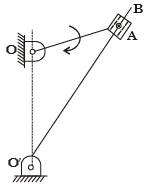Try yourself:A rod of length 1 m is sliding in a corner as shown in figure. At an instant when the rod makes an angle of 60 degrees with the horizontal plane., the velocity of point A on the rod is 1m/ s. The angular velocity of the rod at this instant is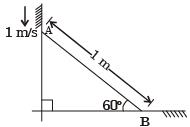Try yourself:The mechanism used in a shaping machine is



Try yourself:The lengths of the links of a 4-bar linkage with revolute pairs only are p, q, r and s units. Given that p < q< r< s. Which of these links should be the fixed one, for obtaining a "double crank" mechanism?



Try yourself:Match the following:
Type of Mechanism
P. Scott - Russel mechanism
Q. Geneva mechanism
R. Off-set slider-crank mechanism
S. Scotch Yoke mechanism

Motion achieved
1. Intermittent motion
2. Quick return motion
3. Simple harmonic motion
4. Straight line motion



Try yourself:An instantaneous configuration of a four-bar mechanism, whose plane is horizontal, is shown in the figure below. At this instant, the angular velocity and angular acceleration of link O2A are ω = 8 rad/s and α = 0, respectively, and the driving torque (τ) is zero. The link O2A is balanced so that its centre of mass falls at O2.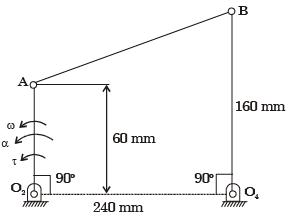Which kind of 4-bar mechanism is O2ABO4?



Try yourself:An instantaneous configuration of a four-bar mechanism, whose plane is horizontal, is shown in the figure below. At this instant, the angular velocity and angular acceleration of link O2A are ω = 8 rad/s and α = 0, respectively, and the driving torque (τ) is zero. The link O2A is balanced so that its centre of mass falls at O2.At the instant considered, what is the magnitude of the angular velocity of O4B?



Try yourself:An instantaneous configuration of a four-bar mechanism, whose plane is horizontal, is shown in the figure below. At this instant, the angular velocity and angular acceleration of link O2A are ω = 8 rad/s and α = 0, respectively, and the driving torque (τ) is zero. The link O2A is balanced so that its centre of mass falls at O2.At the same instant, if the component of the force at Joint A along AB is 30N, then the magnitude of the Joint reaction at O2



Try yourself:In a four-bar linkage, S denotes the shortest link length, L is the longest link length, P and Q are the lengths of other two links. At least one of the three moving links will rotate by 360° if



Try yourself:The input link O2P of a four bar linkage is rotated at 2 rad/s in counter clockwise direction as shown below. The angular velocity of the coupler PQ in rad/s, at an instant when ∠O4O2P = 180°, is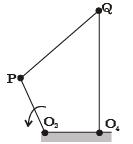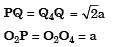Try yourself:A quick return mechanism is shown below. The crank OS is driven at 2 rev/s in counter clockwise direction.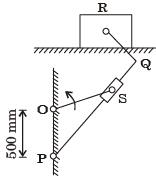If the quick return ratio is 1 : 2, then the length of the crank in mm is



Try yourself:A quick return mechanism is shown below. The crank OS is driven at 2 rev/s in counter clockwise direction.The angular speed of PQ in rev/s when the block R attains maximum speed during forward stroke (stroke with slower speed) is (in rad/s)



Try yourself:A simple quick return mechanism is shown in the figure. The forward to return ratio of the quick return mechanism is 2 :1. If the radius of the crank O, P is 125 mm, then the distance d(in mm) between the crank centre to lever pivot centre point should be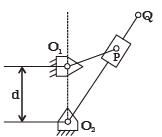Try yourself:For the four-bar linkage shown in the figure, the angular velocity of link AB is 1 rad/s. The length of link CD is 1.5 times the length of link AB. In the configuration shown, the angular velocity of link CD in rad/s is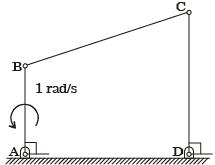Try yourself:A 4-bar mechanism with all revolute pairs has link lengths lf = 20 mm, lin = 40 mm, lco = 50 mm and lout = 60 mm. The suffixes 'f, in', 'co' and 'out' denote the fixed link, the input link, the coupler and output link respectively. Which one of the following statements is true about the input and output links?



Try yourself:Consider a slider crank mechanism with non zero masses and inertia. A constant torque t is applied on the crank as shown in the figure. Which of the following plots best resembles variation of crank angle θ versus time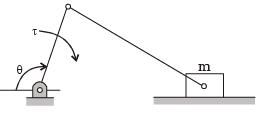Try yourself:For an Oldham coupling used between two shafts, which among the following statements are correct?
I. Torsional load is transferred along shaft axis.
II. A velocity ratio of 1 : 2 between shaft is obtained without using gears.
III. Bending load is transferred transverse to shaft axis.
IV. Rotation is transferred along shaft axis.



Try yourself:In a four bar planar mechanism shown in the figure, AB = 5 cm, AD = 4 cm and DC = 2 cm. In the configuration shown, both AB and DC are perpendicular to AD. The bar AB rotates with an angular velocity of 10 rad/s. The magnitude of angular velocity (in rad/s) of bar DC at this instant is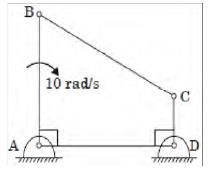Try yourself:The crank of a slider-crank mechanism rotates counter-clock wise (CCW) with a constant angular velocity ω, as shown. Assume the length of the crank to be r.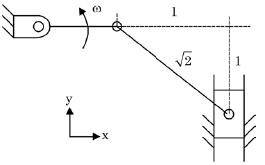Using exact analysis, the acceleration of the slider in the y-direction, at the instant shown, where the crank is parallel to x-axis, is given by



Try yourself:The number of degrees of freedom of a five link plane mechanism with five revolute pairs as shown in the figure is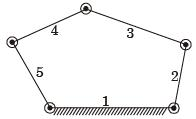Try yourself:Match the following with respect to spatial mechanisms.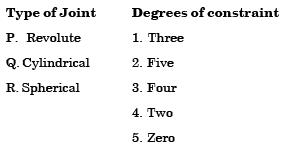Try yourself:The number of degrees of freedom of a planar linkage with 8 links and 9 simple revolute joints is



Try yourself:A planar mechanism has 8 links and 10 rotary joints. The number of degrees of freedom of the mechanism, using Grubler's criterion, is



Try yourself:Mobility of a statically indeterminate structure is



Try yourself:A double parallelogram mechanism is shown in the figure. Note that PQ is a single link. The mobility of the mechanism is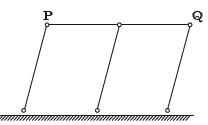Try yourself:The number of degrees of freedom of the linkage shown in the figure is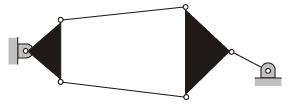Try yourself:The number of degrees of freedom in a planar mechanism having n links and j simple hinge joints is



Offer running on EduRev: Apply code STAYHOME200 to get INR 200 off on our premium plan EduRev Infinity!

## Theory of Machines (TOM)

94 videos|41 docs|28 tests

,

,

,

,

,

,

,

,

,

,

,

,

,

,

,

,

,

,

,

,

,

;